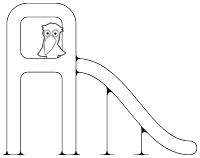### Dinfio Playground

Let's play with Dinfio!

Dinfio Playground is a place where you can find great and fun Dinfio programmes. You can show off your great Dinfio programmes by contributing to the Playground.

You can also try Dinfio online here. So, let's play!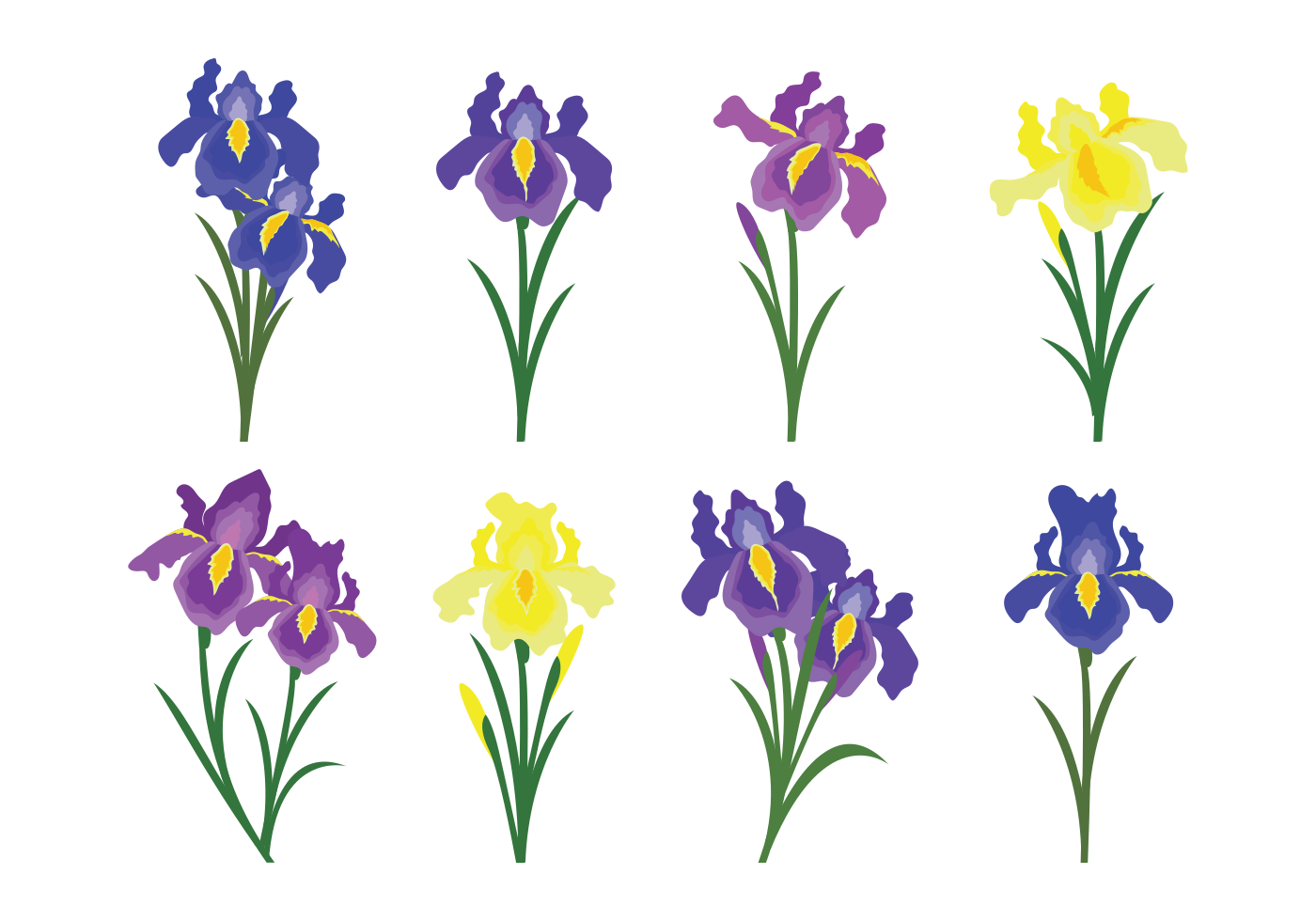Machine Learning: Iris Classification using k-Nearest Neighbours

This is the implementation of k-Nearest Neighbours (kNN) algorithm with Iris as dataset.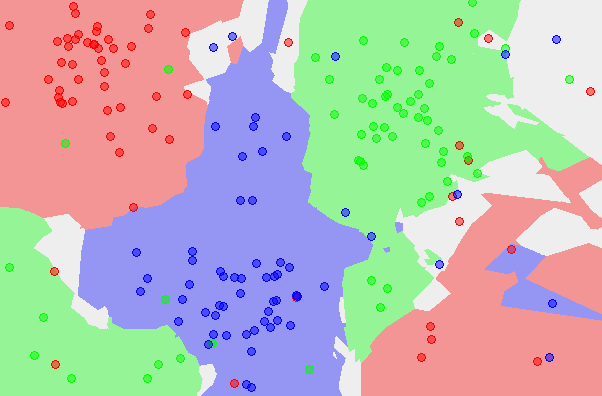Machine Learning: k-Nearest Neighbours

This is the implementation of simple k-Nearest Neighbours (kNN) algorithm with Euclidean distance as the distance metric.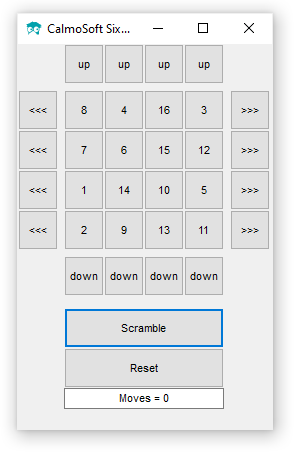CalmoSoft Sixteen Puzzle Game
Author: CalmoSoft

CalmoSoft Sixteen Puzzle Game is a classic 16-puzzle. Sixteen is visually similar to Fifteen but with a different type of move. This time, there is no hole: all 16 squares on the grid contain numbered squares. Your goal is to shift an entire row left or right, or shift an entire column up or down; every time you do that, the tile you shift off the grid re-appears at the other end of the same row, in the space you just vacated. To win, arrange the tiles into numerical order (1, 2, 3, 4 on the top row, 13, 14, 15, 16 on the bottom)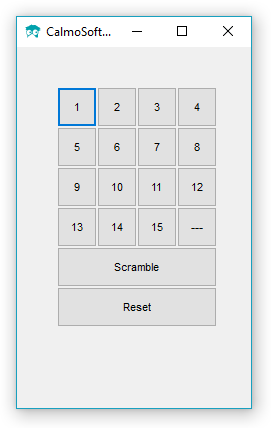CalmoSoft Fifteen Puzzle Game
Author: CalmoSoft

CalmoSoft Fifteen Puzzle Game is a classic 15-puzzle. This is a sliding puzzle that consists of a frame of numbered square tiles in pseudo-random order with one tile missing. The object of the puzzle is to place the tiles in order by making sliding moves that use the empty space.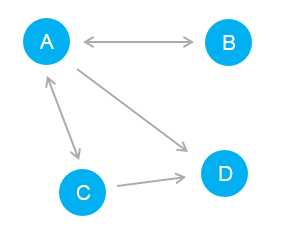Graph Example
Author: Matt Hofmann

This is a graph example using adjacency list. In this example, there are 3 classes: `graph`, `vertex`, and `edge`.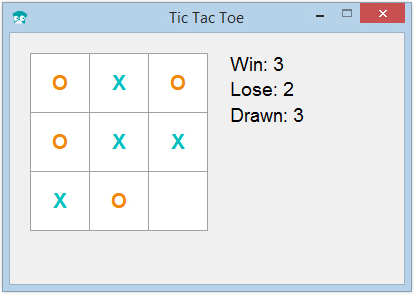3x3 Tic-Tac-Toe Game

This is the Computer vs Human 3x3 Tic-Tac-Toe game example.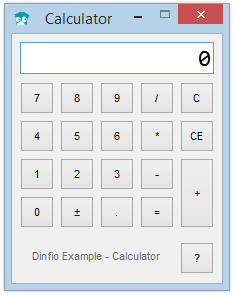Calculator

This basic calculator is an example of object-oriented and GUI programming implementation. This example shows you how your class can be inherited from a GUI object.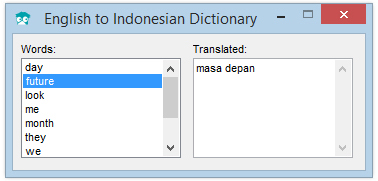Dictionary